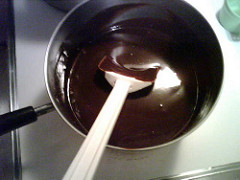# Chemistry Topic 3 – The Mathematics of Formulas and EquationsKenneth Miller
question

Formula mass

The mass of the smallest unit of a compound; the sum of the atomic masses of all the atoms present.
question

Gram formula mass

The formula mass expressed in grams instead of atomic mass units.
question

Mole